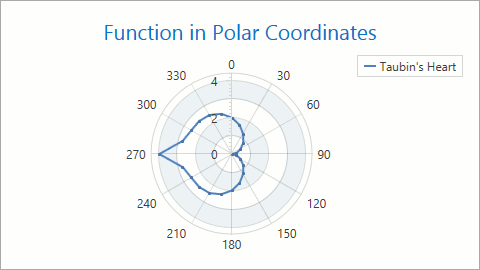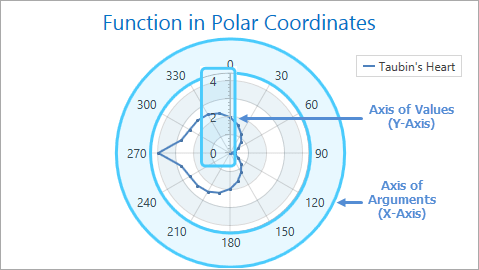20.1
19.2
19.1
18.2
18.1
17.2
The page you are viewing does not exist in version 17.2. This link will take you to the root page.

# 2D Polar Diagram

A 2D Polar Diagram is a diagram in which each point is represented using an angle and the distance from a coordinate origin.The document consists of the following sections:

## Compatible Series Types

The Polar Diagram diagram can only contain the following compatible series types:

## How to Create a Chart with a Polar Diagram

The Polar diagram's circular x-axis shows arguments from 0 to 360 degrees. This axis is available using the PolarDiagram2D.AxisX property.##### NOTE

Note that polar diagram axes do not support additional elements such as constant lines, axis titles and strips.

The following markup demonstrates how to create a chart with the Polar diagram:

``````<dxc:ChartControl>
<!-- Polar diagram's settings. -->
<dxc:PolarDiagram2D RotationDirection="Counterclockwise"
ShapeStyle="Polygon"
DomainBrush="AliceBlue"
DomainBorderBrush="AliceBlue"
StartAngle="180"
LabelsResolveOverlappingMinIndent="5">
<!-- X-axis settings. -->
<dxc:PolarDiagram2D.AxisX>
<dxc:PolarAxisX2D/>
</dxc:PolarDiagram2D.AxisX>
<!-- Y-axis settings. -->
<dxc:PolarDiagram2D.AxisY>
<dxc:PolarAxisY2D/>
</dxc:PolarDiagram2D.AxisY>
<!-- Polar Line series settings. -->
<dxc:PolarLineSeries2D DisplayName="Taubin's Heart">
<dxc:SeriesPoint Argument="0" Value="2.0"/>
<dxc:SeriesPoint Argument="30" Value="1.24"/>
<dxc:SeriesPoint Argument="90" Value="0"/>
<dxc:SeriesPoint Argument="150" Value="1.24"/>
<dxc:SeriesPoint Argument="180" Value="2.0"/>
<dxc:SeriesPoint Argument="210" Value="2.48"/>
<dxc:SeriesPoint Argument="270" Value="4.0"/>
<dxc:SeriesPoint Argument="330" Value="2.48"/>
<dxc:SeriesPoint Argument="360" Value="2.0"/>
</dxc:PolarLineSeries2D>
</dxc:PolarDiagram2D>
</dxc:ChartControl>
``````

The following table lists classes and properties the markup uses:

Class or Property Description
ChartControl The Chart control.
PolarDiagram2D The 2D Polar diagram.
CircularDiagram2D.RotationDirection Specifies the direction in which the diagram's argument axis is drawn.
CircularDiagram2D.ShapeStyle Specifies the diagram's shape style.
CircularDiagram2D.DomainBrush Specifies the brush used to draw the diagram's area.
CircularDiagram2D.DomainBorderBrush Specifies the brush used to draw the diagram's area border.
CircularDiagram2D.StartAngle Specifies an angle that defines the x-axis coordinates' origin position and y-axis deflection.
CircularDiagram2D.LabelsResolveOverlappingMinIndent Specifies the minimum gap between two adjacent series point labels.
PolarDiagram2D.AxisX Gets or sets the x-axis settings.
PolarAxisX2D The polar diagram's circular axis of arguments.
PolarDiagram2D.AxisY Gets or sets the y-axis settings.
PolarAxisY2D The polar diagram's circular axis of values.
PolarLineSeries2D The polar line series.
Series.DisplayName Specifies a series display name.
SeriesPoint A series point.
SeriesPoint.Argument Specifies the series point's argument.
SeriesPoint.Value Specifies the series point's value.

Refer to the code below to access the Polar diagram's settings at runtime:

``````// Cast Diagram to the PolarDiagram2D type.
PolarDiagram2D diagram = chart.Diagram as PolarDiagram2D;
if (diagram != null) {
// Access the diagram's options.
diagram.ShapeStyle = CircularDiagramShapeStyle.Polygon;
}
``````